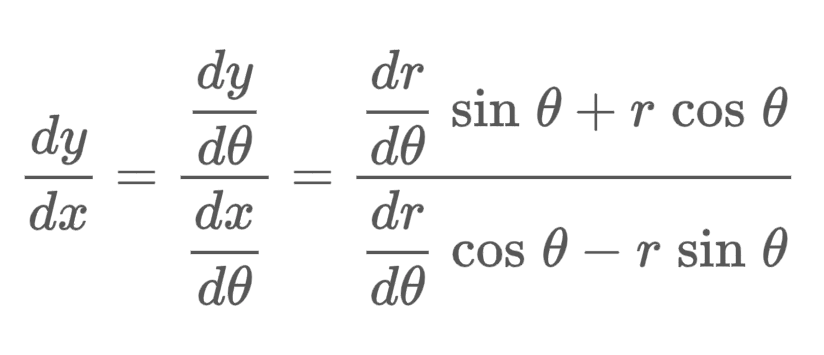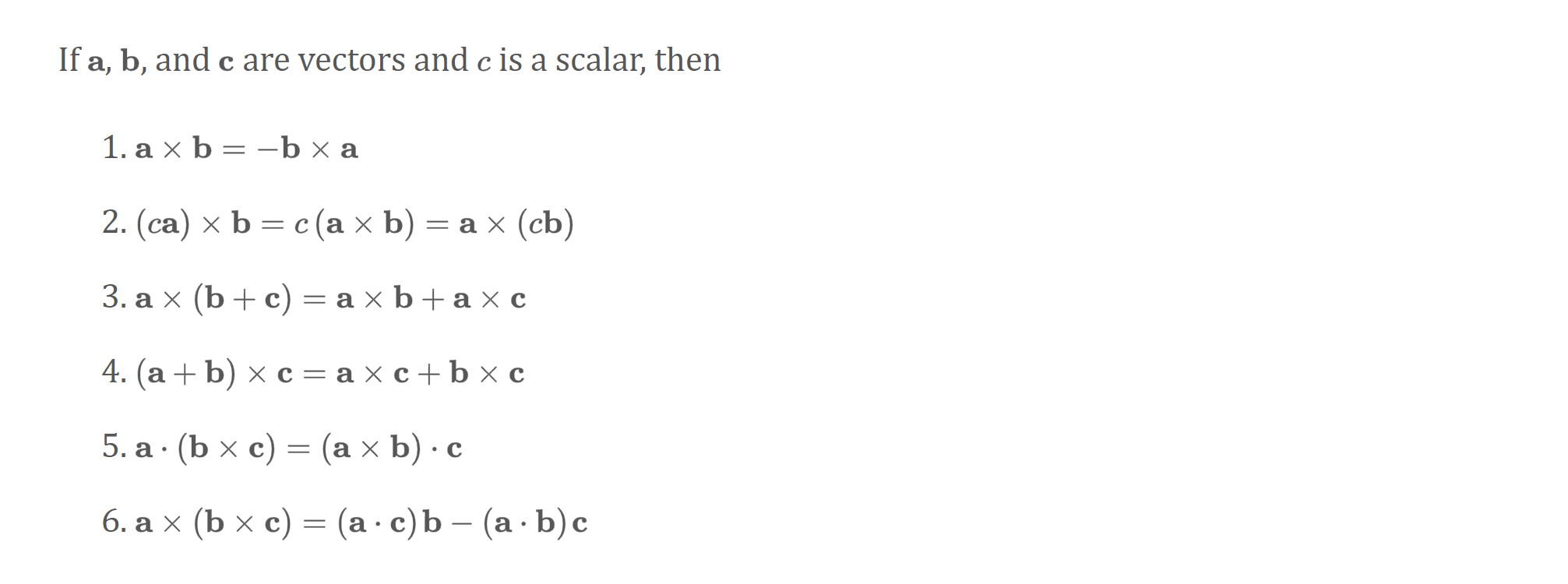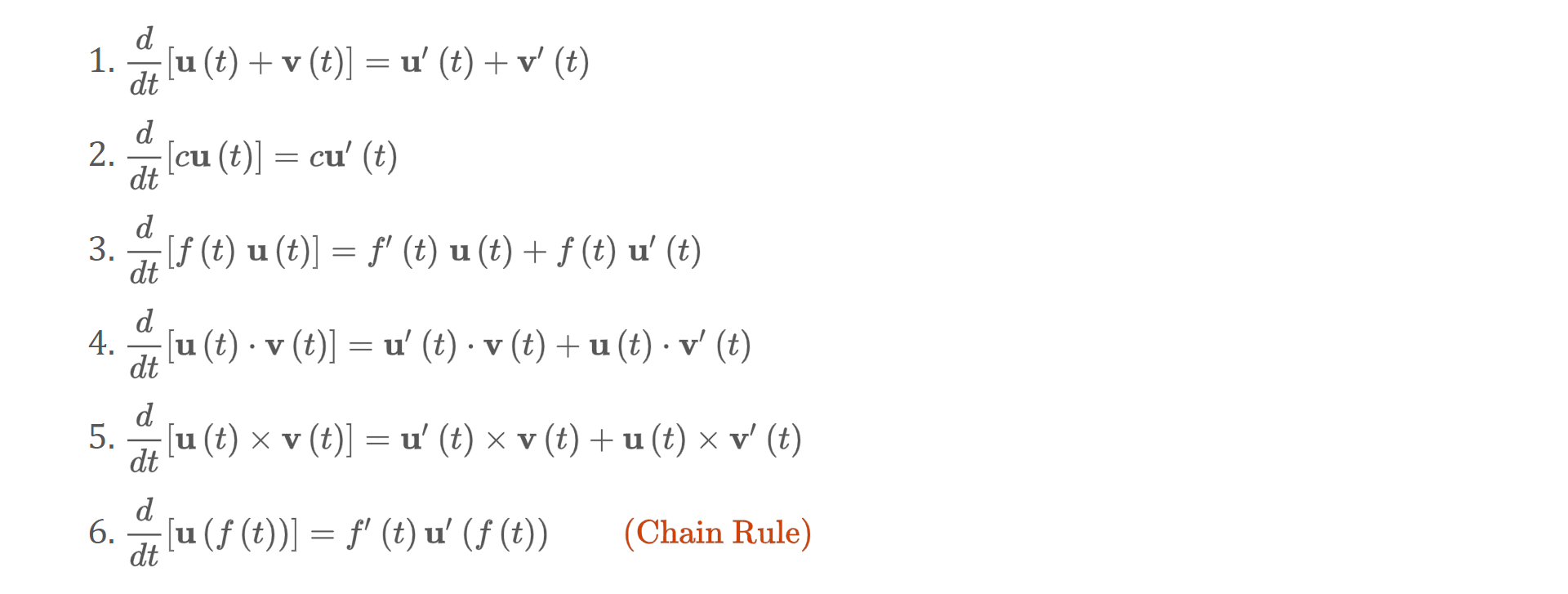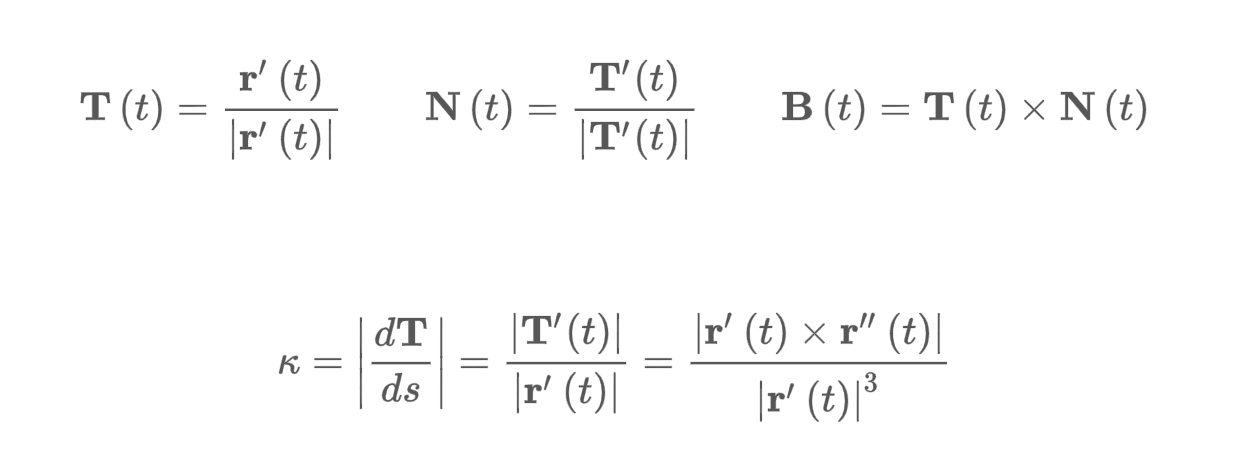# Textbook Notes

MATH 126

## Chapter 10: Parametric Equations and Polar Coordinates

### 10.3: Polar Coordinates

• Polar coordinate system: a point in the plane is the pole. The polar axis is a ray starting from $$0$$.
• Polar coordinates - $$(r, \theta)$$.
• In the polar coordinate system, each point has many representations.
$\cos \theta = \frac{x}{r}, \sin \theta = \frac{y}{r}$ $x = r \cos \theta, y = r \sin \theta$ $r^2 = x^2 y^2; \tan \theta = \frac{y}{x}$

Polar Curves

• Graph of a polar equation - $$r = f(\theta)$$, or $$F(r, \theta) = 0$$.
• ALl points $$P$$ with at least one polar representation $$(r, 0)$$ whose coordinates satisfy the equation.
• Symmetry rules
• If a polar equation is unchanged when $$\theta$$ is negated, the curve is symmetric about the polar axis.
• If the equation is unchanged when $$r$$ is negated or $$\theta$$ replaced with $$\theta + \pi$$, the curve is symmetric about the pole.
• If the equation is unchanged when $$\theta$$ is replaced with $$\pi - \theta$$, the curve is symmetric about the vertical line at $$\theta = \frac{\pi}{2}$$.

Tangents to Polar CUrves

• To find the tangent to $$r = f(\theta)$$, use the following parametric equations:
• $$x = f(\theta) \cos \theta, y = f(\theta) \sin theta$$.## Chapter 12: Vectors and the Geometry of Space

### 12.1: Three-Dimensional Coordinate Systems

• Three axes form a three-dimensional space.
• The three-dimensional space is divided into octants.
• If we drop from $$P$$ to some plane, it is the projection of $$P$$ onto the $$xy$$-plane.
• The Cartesian product $$\mathbb{R} \times \mathbb{R} \times \mathbb{R} = {(x, y, z) \| x, y, z \in \mathbb{R}}$$ is the set of all ordered triples of real numbers; denoted $$\mathbb{R}^3$$.

#### Surfaces

• If $$k$$ is constant, then:
• $$x = k$$ represents a plane parallel to the $$yz$$-plane
• $$y = k$$ represents a plane parallel to the $$xz$$-plane
• $$z = k$$ represents a plane parallel to the $$xy$$-plane
• Circles and cylinders, free restirction over axes

#### Distance and Spheres

The distance formula $$\|P_1P_2\|$$ between two points $$P_1 (x_1, y_1, z_1)$$ and $$P_2 (x_2, y_2, z_2)$$ is

$\|P_1P_2\| = \sqrt{(x_2 - x_1)^2 + (y_2 - y_1)^2 + (z_2 - z_1)^2}$
• This formula is a natural extension of the Pythagorean theorem in two dimensions.
• The equation of a sphere with center $$C (h, k, l)$$ and radius $$r$$ is
$(x - h)^2 + (y - k)^2 + (z - l)^2 = r^2$

### 12.2: Vectors

• Vector: used to indicate a quantity that has both magnitude and direction. Often represented by an arrow or directed line segment. Vectors are denoted as $$\vec{v}$$.
• A displacement vector $$v$$ has initial point $$A$$ and terminal point $$B$$, which can be notated as $$\mathbf{v} = \vec{AB}$$.
• To combine vectors, simply add the changes.
• You can multiply a vector by a scalar $$c$$.

Components

• If we place the initial point of a vector $$a$$ at the origin of a rectangular coordinate system, the terminal point of $$a$$ has a coordinate of the form $$(a_1, a_2)$$ or $$(a_1, a_2, a_3)$$.
• Coordinates are the components of $$\mathbf{a}$$. We can write these as
$\mathbf{a} = \langle a_1, a_2 \rangle$ $\mathbf{a} = \langle a_1, a_2, a_3 \rangle$
• This represents a vector rather than a point.
• Different representations of a vector have the same direction and magnitude but may be in different positions. The position with the beginning point at the origin is the posiiton vector of a point.
• The magnitude or length of a vector is the length of any of its representations, and is denoted as $$\| \vec{v} \|$$.
• An $$n$$ dimensional vector is an ordered $$n$$-tuple.
• Basis vectors:
• $\vec{i} = \langle 1, 0, 0, \rangle$
• $\vec{i} = \langle 0, 1, 0, \rangle$
• $\vec{i} = \langle 0, 0, 1, \rangle$
• Any vector can be expressed as a linear sum of basis vectors.
• Unit vector: a vector whose length is 1. In general if $$\vec{a} \neq 0$$, then the unit vector that has the same direction as $$\vec{a}$$ is $$\vec{u} = \frac{\vec{a}}{\|\vec{a}\|}$$.

Applications

• Vectors are useful in many aspects of physics and engineering.
• Force is represented by vector because it obht has magnitude and direction.

### 12.3: The Dot Product

• Can we multiply two vectors such that the product is a useful quantity?

If $$\vec{a} = \langle a_1, a_2, a_3 \rangle$$ and $$\vec{b} = \langle b_1, b_2, b_3 \rangle$$, then the dot product $$\vec{a} \cdot \vec{b}$$ is

$\vec{a} \cdot \vec{b} = a_1 b_1 + a_2 b_2 + a_3 b_3$
• The result of a dot product is not a vector, but a scalar. The dot produce is sometimes called the scalar product or inner product.

• Properties of the dot product:
1. $$\vec{a} \cdot \vec{a} = |\vec{a}|^2 2. $\vec{a} \cdot \vec{b} = \vec{b} \cdot \vec{a}$ 3. $0 \cdot \vec{a} = 0$ 4. $\vec{a} \cdot (\vec{b} + \vec{c}) = \vec{a} \cdot \vec{b} + \vec{a} \cdot \vec{c}$ 5. $(c\vec{a}) \cdot \vec{b} = c(\vec{a} \cdot \vec{b}) = \vec{a} \cdot (c\vec{b})$ • The dot product can be interpreted in terms of the angle between $$\vec{a}$$ and $$\vec{b}$$: If $$\theta$$ is the angle between the vectors $$\vec{a}$$ and $$\vec{b}$$, then $\vec{a} \cdot \vec{b} = \|\vec{a} \| \| \vec{b} \| \cos \theta$ If $$\theta$$ is the angle between the nonzero vectors $$\vec{a}$$ and $$\vec{b}$$, then $\cos \theta = \frac{\vec{a} \cdot \vec{b}}{\| \vec{a} \| \| \vec{b} \|}$ • Two nonzero vectors are perpendicular/orthogonal if the angle between them is $$\theta = \frac{\pi}{2}$$. Two nonzero vectors ar eorthogonal if and only if their dot product is 0. Direction Angles and Direction Cosines • Direction angles of a nonzero vector are the angles $$\alpha, \beta, \gamma$$ that a vector makes with the positive axes. • The cosines of the direction angles are the direction cosines. $\cos \alpha = \frac{a_1}{\|\vec{a}\|}$ $\cos \beta = \frac{a_2}{\|\vec{a}\|}$ $\cos \gamma = \frac{a_3}{\|\vec{a}\|}$ • We can square expressions and see the following: $\cos^2 \alpha + \cos^2 \beta + \cos^2 \gamma = 1$ • We can also write the following: $\vec{a} = \langle a_1, a_2, a_3 \rangle = \langle \| \vec{a} \| \cos \alpha, \| \vec{a} \| \cos \beta, \| \vec{a} \| \cos \gamma \rangle = \| \vec{a} \| \langle \cos \alpha, \cos \beta, \cos \gamma \rangle$ • Dividing both sides by $$\| \vec{a} \|$$, we see that the direction cosines of $$\alpha$$ are the components of the unit vector in the direction of $$\vec{a}$$. Projections • Consider $$\vec{PQ}$$ $$\mathbf{a}$$ and $$\vec{PR}$$ $$\mathbf{b}$$ with the same initial point $$\vec{P}$$. If $$S$$ is the foot of the perpendicular from $$R$$ to the line containing $$\vec{PQ}$$, then the vector with representation $$\vec{PS}$$ is the vector projection of $$\mathbf{b}$$ onto $$\mathbf{a}$$, denoted by $$\text{proj}_a \mathbf{b}$$. • Think of this as the shadow of $$\mathbf{b}$$. • Scalar projbection of $$\mathbf{b}$$ onto $$\mathbf{a}$$ (the component of $$\mathbf{b}$$ along $$\mathbf{a}$$) is the signed magnitude of the vector projection, which is $$\| \mathbf{b} \| \cos \theta$$, where $$\theta$$ is the angle between $$\mathbf{a}$$ and $$\mathbf{b}$$. This is denoted as $$\text{comp}_a \mathbf{b}$$; this is negative if $$\frac{\pi}{2} < \theta \le \pi$$. $\mathbf{a} \cdot \mathbf{b} = \| \mathbf{a} \| \| \mathbf{b} \| \cos \theta = \| \mathbf{a} \| (\| \mathbf{b} \| \cos \theta )$ • The dot product of two vectors can be interpreted as the length of one multiplied by the scalar projection of the other vector upon the first vector. Calculating work • The work done by a constant force $$\vec{F}$$ is $$\vec{F} \cdot \vec{D}$$, where $$\vec{D}$$ is the displacement vector. ### 12.4: The Cross Product • Given two nonzero vectors, it is very useful to find a nonzero vector that is perpendicular to both vectors. • The cross product of $$\vec{a} = \langle a_1, a_2, a_3 \rangle$$ and $$\vec{b} = \langle b_1, b_2, b_3 \rangle$$ is $\vec{a} \times \vec{b} = \langle a_2 b_3 - a_3 b_2, a_3 b_1 - a_1 b_3, a_1 b_2 - a_2 b_1 \rangle$ • The cross product/vector product is only defined when $$\vec{a}$$ and $$\vec{b}$$ are three-dimensional. • Determinant interpretation of the cross-product. • The vector $$\vec{a} \times \vec{b}$$ is orthogonal to both $$\vec{a}$$ and $$\vec{b}$$. • The right-hand rule gives the direction of $$\vec{a} \times \vec{b}$$. • If $$\theta$$ is the angle between $$\vec{a}$$ and $$\vec{b}$$, then $$\| \vec{a} \times \vec{b} \| = \| \vec{a} \| \| \vec{b} \| \sin \theta$$. • Geometric characterization: $$\vec{a} \times \vec{b}$$: a vector perpendicular both to $$\vec{a}$$ and $$\vec{b}$$. • Two nonzero vectors $$\vec{a}$$ and $$\vec{b}$$ are parallel if and onlny if $$\vec{a} \times \vec{b} = 0$$. • The length of the crossproduct $$\vec{a} \times \vec{b}$$ is equal to the area of the parallelogram formed by $$\vec{a}$$ and $$\vec{b}$$. • The cross-product is not commutative. $$\vec{i} \times \vec{j} \neq \vec{j} \times \vec{i}$$. The associative law does not hold either.Triple Products • The product $$\vec{a} \cdot (\vec{b} \times \vec{c})$$ - scalar triple product of the vectors $$\vec{a}$$, $$\vec{b}$$, and $$\vec{c}$$. • Parallelepiped volume $$V$$ is the magnitude of the scalar triple: $V = Ah = \| \vec{b} \times \vec{c} \| \| \vec{a} \| \| \cos \theta \| = \| \vec{a} \cdot (\vec{b} \times \vec{c} ) \|$ • If the scalar product is 0, the vectors lie on the same plane and are coplanar. ### 12.5: Equations of Lines and Planes Let $$P(x, y, z)$$ be an arbitrary point on a line $$L$$. Let $$\vec{r}_0$$ and $$\vec{r}$$ be the position vectors of $$P_0$$ and $$P$$. The following is true for some vector $$\vec{a} = \vec{P_0 P}$$ and some scalar $$t$$. $\vec{r} = \vec{r}_0 + t \vec{v}$ This is the vector equation of the line. We can expand the vector equation of a line, and we obtain the parameteric equations derived from identifying the components of the result of the vector equation. $x = x_0 = at$ $y = y_0 + bt$ $z = z_0 + ct$ The vector equation and parameteric equations of a line are not unique; we can change the point, parameter, or choose a different parallel vector. This will change the line. • Symmetric equations: derived from solving each of the parameter equations for $$t$$, then setting the expressions all equal to each other. • You can set a limitation on $$t$$ to express only a certain line segment. • The line segment from $$\vec{r}_0$$ to $$\vec{r}_1$$ is given by the vector equation $\vec{r} = (1 -t ) \vec{r}_0 + t \vec{r}_1, 0 \le t \le 1$ A plane in space is more difficult to describe. A plane in space is determined by a point $$P_0$$, a vector $$\vec{n}$$ orthogonal to the plane. Let $$\vec{r}_0$$ and $$\vec{r}$$ be the position vectors of $$P_0$$ and $$P$$. $$\vec{r} - \vec{r}_0$$ is orthogonal to $$\vec{n}$$. $\vec{n} \cdot (\vec{r} - \vec{r}_0) = 0 \implies \vec{n} \cdot \vec{r} = \vec{n} \cdot \vec{r}_0$ Scalar equation for the plane: write $\vec{n} = \langle a, b, c \rangle$ $\vec{r} = \langle x, y, z \rangle$ $\vec{r}_0 = \langle x_0, y_0, z_0 \rangle$ This simplifies into $a (x - x_0) + b(y - y_0) + c(z - z_0) = 0$ We can rewrite the equation as such: $ax + by + cz + d = 0$ Here, $$d = -(ax_0 + by_0 + cz_0)$$. This is the linear equation in $$\mathbb{R}^3$$. ### 12.6: Surfaces • Cylinders and quadric surfaces. • Traces/cross-sections - curves of intersection on the surface with planes paralle to the coordinate planes. Cylinders • Consists of all lines that are parallel to a given line and pass through a given plane curve. • Parabolic cylinder - made up of infinitely many shifted copies of the same parabola. Quadric Surfaces • A quadric surface is the graph of a second degree equation in three variables. $Ax^2 + By^2 + Cz^2 + Dxy + Eyz + Fxz + Gx + Hy + Iz + J = 0$ • To sketch an image, find how traces scale in each dimension. ## Chapter 13: Vector Functions ### 13.1: Vector Functions and Space Curves • Vector-valued function/vector function: a function whose domain is a set of real numbers and whose range is a set of vectors. • Each number $$t$$ in the domain of $$\mathbf{r}$$ has a unique vector in $$\mathbf{V}_3$$. • If $$\{f(t), g(t), h(t)}$$ are components of the vector$$\mathbf{r}44, we have:
$\mathbf{r}(t) = \langle f(t), g(t), h(t) \rangle = f(t) \vec{i} + g(t) \vec{j} + h(t) \vec{k}$

Limits and Continuity.

• The limit of a vector function is defined by taking the limits of the component functions.
$\lim_{t\to a} \mathbf{r}(t) = \langle \lim_{t\to a} f(t), \lim_{t\to a} g(t), \lim_{t\to a} h(t) \rangle$
• Taking the limit can be interpreted as saying that the length and direction of the vector $$\mathbf{r}$$ approach the length and direction of the vector $$\vec{L}$$ (where $$\vec{L}$$ is the result of the limit.
• A vector function is continuous at $$a$$ if $$\lim_{t\to a} \mathbf{r}(t) = \mathbf{r}(a)44. • The component functions of a vector function must all be continuous at $$a$$ for the vector function itself to be considered continuous at $$\vec{a}$$. Space curves. • Set $$C$$ of all points $$(x, y, z)$$ with $$x = f(t), y=g(t), z = h(t)$$, the space curve is derived by tracing $$t$$ through an interval $$I$$. • The equations are the parametric equations of $$C$$; $$t$$ is the parameter. • Any continuous vector defines space curve $$C$$ traced out by moving the tip of the moving vector $$\mathbf{r}(t)$$. • Plane curves can be represented in vector notation. The curve given by the parametric equations $$x = t^2 - 2t$$ and $$y = t + 1$$ can be described via $\mathbf{r}(t) = \langle t^2 - 2t, t + 1 \rangle$ • Helix: given by $$x = \cos t, y = \sin t, z = t$$ ### 13.2: Derivatives and Integrals of Vector Functions Derivatives. • The derivative of a vector function $$\mathbf{r}$$ is dfined similarly for real-valued functions. Take the derivative of the element-wise component functions. • The second derivaitv eof avector function is the derivative of $$\mathbf{r}$$. Differentiation Rules • Differentiation formulas for real-valued functions have counterparts for vector-valued functions.Integrals. • You can integrate component-wise, like normal. ### 13.3: Arc Length and Curvatutre $L = \int_a^b \int{f'(t)^2 + g'(t)^2 + g'(t)^2}$ $L = \int_a^b \| r'(t) \| dt$ • $$r(t)$$ is the position vector, $$r'(t)$$ is the velocity vector, $$\| r'(t) \|$$ is the speed. To compute distance traveled, integrate speed. The Arc Length Function • Arc length function $$s$$: defined as follows for some $$a$$: $s(t) = \int_a^t \| \vec{r}'(u) \| du$ • We see that $$\frac{ds}{dt} = \| \vec{r} '(t) \|$$ • It is useful to parametrize a curve w.r.t. arc length - arc length arises naturally from the curve shape and is not coordinate-dependent. • Solve for $$t$$ as a function of $$s$$, then reparametrize in terms of $$s$$. Curvature • A parametrization is smooth on an interval if the derivative is continuous and $$\vec{r}'(t) \neq 0$$. A curve is smooth if it has a smooth parametrization. Smooth curves have no sharp corners; the tangent vector turns continuously. • Curvature - measure of how quickly the curve changes direction. • The curvature of a curve is $$\| \frac{d\vec{T}}{ds} \| = \| \frac{\vec{T}'(t)}{\vec{r}'(t)} \|$$, where $$\vec{T}$$ is the unit tangent vector. • The curvature of the curve given by a vector function $$\vec{r}$$ is $\frac{\| \vec{r}'(t) \times \vec{r} ''(t) \|}{\| \vec{r}'(t) \| ^3}$ The Normal and Binormal Vectors • Many vectors are orthogonal to the unit tangent vector $$\vec{T}(t)$$. Note that $$\vec{T}(t) \cdot \vec{T}'(t) = 0$$, so $$\vec{T}'(t)$$ is orthogonal to $$\vec{T}(t)$$. • At any point where $$\kappa \neq 0$$, we can define the principal unit normal vector/unit normal vector $$\vec{N}(t) = \frac{\vec{T}'(t)}{\| \vec{T}'(t) \|$$. • The unit normal vector indicates the direction in which the curve is turning at each point. • $$\vec{B}(t) = \vec{T}(n) \times \vec{N}(t)$$ - binormal vector. Is a unit vector orthogonal to $$\vec{T}(t)$$ and$$\vec{N}(t)44.
• Normal plane - plane spanned by the normal and binormal vectors. Consists of all lines orthogonal to the tangent line
• Osculating plane spanned by $$\vec{T}$$ and $$\vec{N}$$. Plane that comes closest to containing the part of the curve near $$\vec{P}$$.
• Osculating circle/circle of curvature of $$C$$ at $$P$$. Shares the same tangent, normal, and curvature at $$P$$.### 13.4: Motion in Space

• Tangent and normal vectors can be used in physics to study velocity and acceleration along a space curve.
• Velocity vector $$\vec{v}(t) = \vec{r}'(t)$$.
• Speed is the magnitude of the velocity vector.
• Acceleration is the derivative of velocity: $$\vec{a}(t) = \vec{v}'(t)$$.
• Vector integrals allow us to recover velocity when acceleration is known and position when velocity is known.
$\vec{v}(t) = \vec{v}(t_0) + \int_{t_0}^t \vec{a}(u) du$ $\vec{r}(t) = \vec{r}(t_0) + \int_{t_0}^t \vec{v}(u) du$
• Newton’s Second Law of Motion: a force $$\vec{F}(t)$$ acts on an object of mass $$m$$ produces an acceleration $$\vec{a}(t)$$. The following is true:
$\vec{F}(t) = m\vec{a}(t)$

Tangent and Normal Components of Acceleration

• Acceleration can be separated into the direction of the tangent and the direction of the normal.

### 15.4: Applications of Double Integrals

Density and Mass

• We can approximate the mass of an object by integrating over the surface.
• We can measure total charge by integrating over the individual charges at each instance.

Moments and Centers of Mass

• We can consider a lamina with uniform density using double integrals.
$M_x = \int \int_D y \rho (x, y) dA$ $M_y = \int \int_D x \rho (x, y) dA$ $\bar{x} = \frac{M_y}{m}, \bar{y} = \frac{M_x}{m}$ $m = \int \int_D \rho (x, y) dA$

Moment of Inertia

• Second moment/moment of inertia: $$mr^2$$, where $$m$$ is the pass of the particle and $$r$$ is the distance from the particle to the axis.
• Apply to a lamina with density function $$\rho(x, y)$$.

Moment of inertia of the lamina about the $$x$$-axis:

$I_x = \int \int_D y^2 \rho(x, y) dA$

Moment of inertia of the lamina about the $$y$$-axis:

$I_y = \int \int_D x^2 \rho(x, y) dA$

Moment of inertia of the lamina about the origin/polar moment of inertia:

$I_0 = \int \int_D (x^2 + y^2) \rho(x, y) dA$ $I_0 = I_x + I_y$